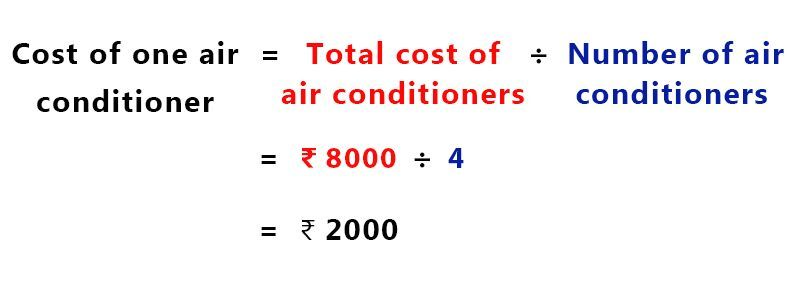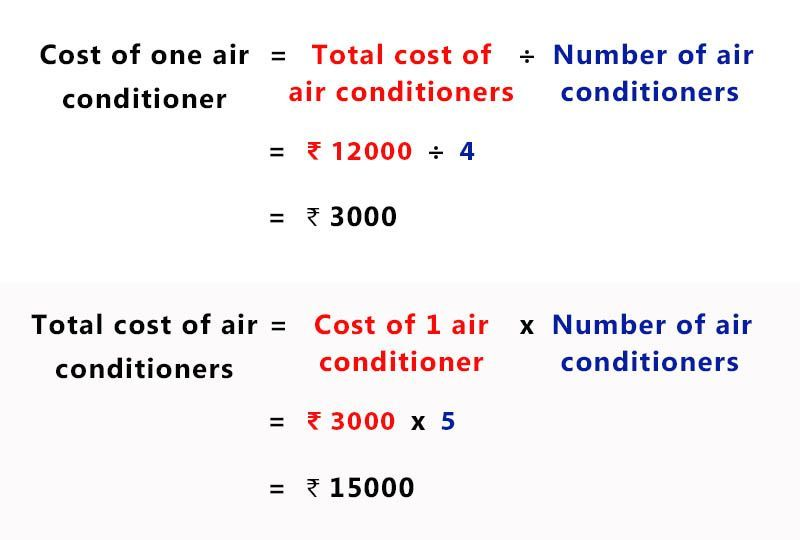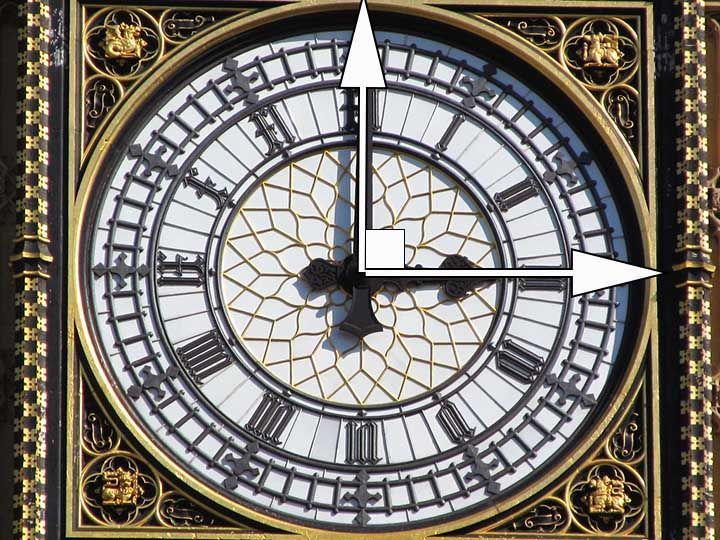# Unitary Method

Unitary Method is used in finding the relations between different quantities given we know one relation. There are 3 rules in this method.

1. Finding the cost of many quantities knowing the cost of 1 quantity of the same type.2. Finding the cost of 1 quantity knowing the cost of many quantities of the same type.3. Finding the cost of many quantities knowing the cost of some number of quantities of the same type.# Access All Content

## More from Grade 6 Math# Types of Lines

All you need to know about lines, parallel, perpendicular and intersecting lines. Along with all the examples from the world around you.# Types of Angles

Learn about the different types of angles acute, right, obtuse and more through exciting facts from the Wonders of the World.# Celsius to Fahrenheit

Learn how to check the temperature, anytime and anywhere.